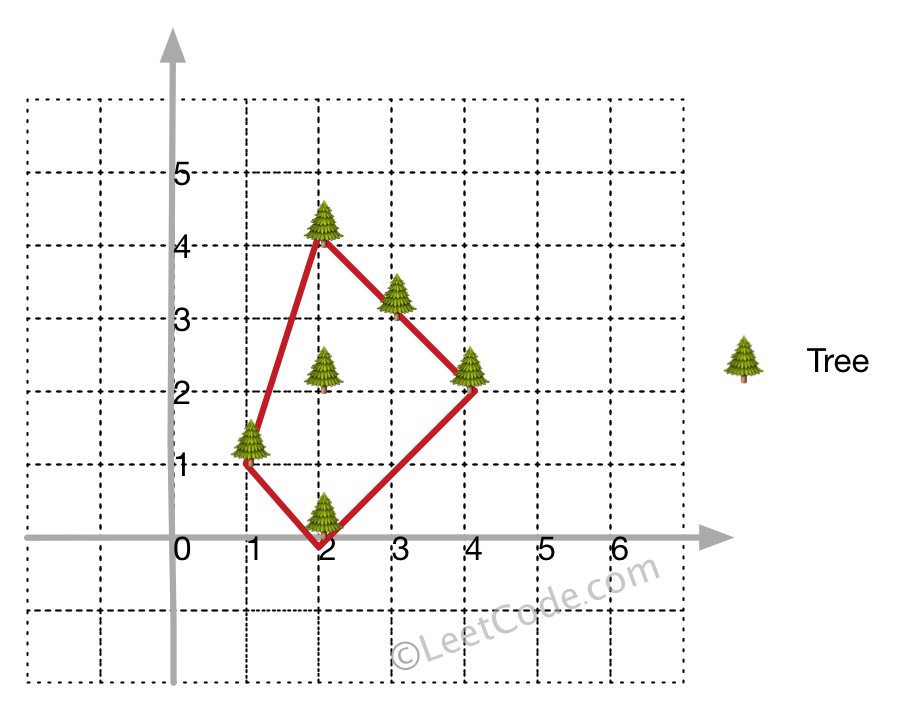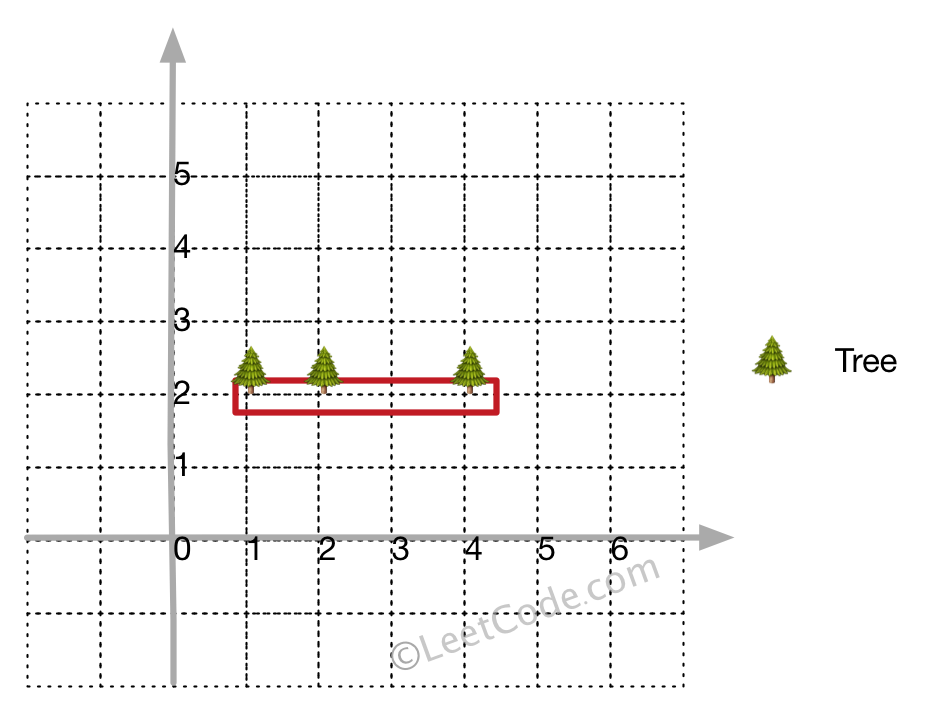##### Welcome to Subscribe On Youtube

Formatted question description: https://leetcode.ca/all/587.html

# 587. Erect the Fence

Hard

## Description

There are some trees, where each tree is represented by (x,y) coordinate in a two-dimensional garden. Your job is to fence the entire garden using the minimum length of rope as it is expensive. The garden is well fenced only if all the trees are enclosed. Your task is to help find the coordinates of trees which are exactly located on the fence perimeter.

Example 1:

Input: [[1,1],[2,2],[2,0],[2,4],[3,3],[4,2]]

Output: [[1,1],[2,0],[4,2],[3,3],[2,4]]

Explanation:Example 2:

Input: [[1,2],[2,2],[4,2]]

Output: [[1,2],[2,2],[4,2]]

Explanation:Even you only have trees in a line, you need to use rope to enclose them.

Note:

1. All trees should be enclosed together. You cannot cut the rope to enclose trees that will separate them in more than one group.
2. All input integers will range from 0 to 100.
3. The garden has at least one tree.
4. All coordinates are distinct.
5. Input points have NO order. No order required for output.
6. Input types have been changed on April 15, 2019. Please reset to default code definition to get new method signature.

## Solution

Use Jarvis algorithm to find the convex hull. Starting from the leftmost point, consider all the points on the fence in counterclockwise direction. For each point p, find point q that is the closest to p in the counterclockwise direction, and point q is on the fence. When the start point is met, the fence is completed.

• class Solution {
public int[][] outerTrees(int[][] points) {
int length = points.length;
if (length < 4)
return points;
Arrays.sort(points, new Comparator<int[]>() {
public int compare(int[] point1, int[] point2) {
if (point1 != point2)
return point1 - point2;
else
return point1 - point2;
}
});
List<int[]> hullList = new ArrayList<int[]>();
Set<String> hullSet = new HashSet<String>();
int p = 0;
do {
int q = (p + 1) % length;
for (int i = 0; i < length; i++) {
if (orientation(points[p], points[i], points[q]) < 0)
q = i;
}
for (int i = 0; i < length; i++) {
if (i == p || i == q)
continue;
if (orientation(points[p], points[i], points[q]) == 0 && isBetween(points[i], points[p], points[q])) {
}
}
p = q;
} while (p != 0);
int hullLength = hullList.size();
int[][] hullArray = new int[hullLength];
for (int i = 0; i < hullLength; i++) {
int[] hull = hullList.get(i);
for (int j = 0; j < 2; j++)
hullArray[i][j] = hull[j];
}
return hullArray;
}

public int orientation(int[] point1, int[] point2, int[] point3) {
return (point2 - point1) * (point3 - point2) - (point3 - point2) * (point2 - point1);
}

public boolean isBetween(int[] point, int[] point1, int[] point2) {
boolean xBetween = point >= point1 && point <= point2 || point <= point1 && point >= point2;
boolean yBetween = point >= point1 && point <= point2 || point <= point1 && point >= point2;
return xBetween && yBetween;
}
}

• class Solution:
def outerTrees(self, trees: List[List[int]]) -> List[List[int]]:
def cross(i, j, k):
a, b, c = trees[i], trees[j], trees[k]
return (b - a) * (c - b) - (b - a) * (c - b)

n = len(trees)
if n < 4:
return trees
trees.sort()
vis = [False] * n
stk = 
for i in range(1, n):
while len(stk) > 1 and cross(stk[-2], stk[-1], i) < 0:
vis[stk.pop()] = False
vis[i] = True
stk.append(i)
m = len(stk)
for i in range(n - 2, -1, -1):
if vis[i]:
continue
while len(stk) > m and cross(stk[-2], stk[-1], i) < 0:
stk.pop()
stk.append(i)
stk.pop()
return [trees[i] for i in stk]

############

# Definition for a point.
# class Point(object):
#     def __init__(self, a=0, b=0):
#         self.x = a
#         self.y = b

class Solution(object):
def outerTrees(self, points):
"""
:type points: List[Point]
:rtype: List[Point]
"""
if len(points) == 1:
return points

def direction(p, q, r):
return ((p.x - r.x) * (q.y - r.y) - (p.y - r.y) * (q.x - r.x))

points.sort(key=lambda x: (x.x, x.y))
upper = []
lower = []
for point in points:
while len(lower) >= 2 and direction(lower[-2], lower[-1], point) < 0:
lower.pop()
lower.append(point)

for point in reversed(points):
while len(upper) >= 2 and direction(upper[-2], upper[-1], point) < 0:
upper.pop()
upper.append(point)

return list(set(upper[1:] + lower[1:]))


• class Solution {
public:
vector<vector<int>> outerTrees(vector<vector<int>>& trees) {
int n = trees.size();
if (n < 4) return trees;
sort(trees.begin(), trees.end());
vector<int> vis(n);
vector<int> stk(n + 10);
int cnt = 1;
for (int i = 1; i < n; ++i) {
while (cnt > 1 && cross(trees[stk[cnt - 1]], trees[stk[cnt - 2]], trees[i]) < 0) vis[stk[--cnt]] = false;
vis[i] = true;
stk[cnt++] = i;
}
int m = cnt;
for (int i = n - 1; i >= 0; --i) {
if (vis[i]) continue;
while (cnt > m && cross(trees[stk[cnt - 1]], trees[stk[cnt - 2]], trees[i]) < 0) --cnt;
stk[cnt++] = i;
}
vector<vector<int>> ans;
for (int i = 0; i < cnt - 1; ++i) ans.push_back(trees[stk[i]]);
return ans;
}

int cross(vector<int>& a, vector<int>& b, vector<int>& c) {
return (b - a) * (c - b) - (b - a) * (c - b);
}
};

• func outerTrees(trees [][]int) [][]int {
n := len(trees)
if n < 4 {
return trees
}
sort.Slice(trees, func(i, j int) bool {
if trees[i] == trees[j] {
return trees[i] < trees[j]
}
return trees[i] < trees[j]
})
cross := func(i, j, k int) int {
a, b, c := trees[i], trees[j], trees[k]
return (b-a)*(c-b) - (b-a)*(c-b)
}
vis := make([]bool, n)
stk := []int{0}
for i := 1; i < n; i++ {
for len(stk) > 1 && cross(stk[len(stk)-1], stk[len(stk)-2], i) < 0 {
vis[stk[len(stk)-1]] = false
stk = stk[:len(stk)-1]
}
vis[i] = true
stk = append(stk, i)
}
m := len(stk)
for i := n - 1; i >= 0; i-- {
if vis[i] {
continue
}
for len(stk) > m && cross(stk[len(stk)-1], stk[len(stk)-2], i) < 0 {
stk = stk[:len(stk)-1]
}
stk = append(stk, i)
}
var ans [][]int
for i := 0; i < len(stk)-1; i++ {
ans = append(ans, trees[stk[i]])
}
return ans
}